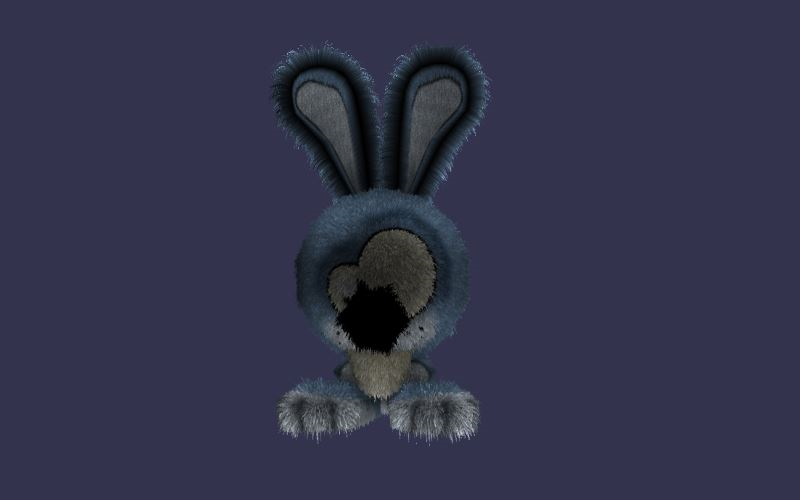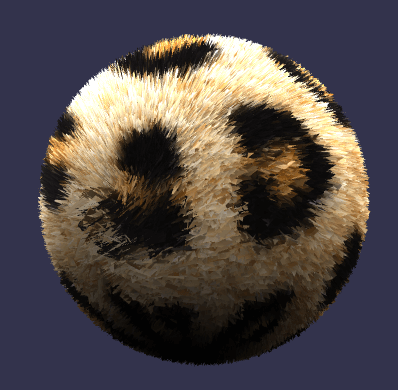# Fur MaterialFur material can be found here: https://cdn.babylonjs.com/materialsLibrary/babylon.furMaterial.js

## Using the High Level mode

A demo can be found at: PG: Fur Material

Fur materials have always been subjects of a lot of theories and conferences with multiple implementations thanks to multiple technologies. Here, with WebGL, we decided to choose one of these implementations, not hard to use and pretty smart (with performances) with simple models

First, activate the high level (activated by default):

javascript
furMaterial.highLevelFur = true;

That's all. Now, the most difficult part should be to configure the shells and the fur texture to create the fur effect. Indeed, you'll have to draw several times the same mesh with an offset (computed in the effect) to create the illusion of fur. Hopefully, there is a function that creates and returns the shells:

javascript
// Generate a fur texture (internally used), working like a noise texture, that will be shared between all the shellsvar furTexture = BABYLON.FurMaterial.GenerateTexture("furTexture", scene);
furMaterial.furTexture = furTexture;myMesh.material = furMaterial;
var quality = 30; // Average quality
// Create shellsvar shells = BABYLON.FurMaterial.FurifyMesh(myMesh, quality);

It is now working! The function "BABYLON.FurMaterial.FurifyMesh" returns an array of "BABYLON.Mesh" that you can dispose later. The first element is the mesh you used as the source mesh (myMesh here):

javascript
for (let i = 0; i < shells.length; i++) {  shells[i].material.dispose();  shells[i].dispose();}

You can customize the high level fur rendering thanks to some properties:

javascript
allFurMaterials.furSpacing = 2; // Computes the space between shells. In others words, works as the fur height
javascript
allFurMaterials.furDensity = 20; // Computes the fur density. More the density is high, more you'll have to zoom on the model
javascript
allFurMaterials.furSpeed = 100; // Divides the animation of fur in time according to the gravity
javascript
// Compute the gravity followed by the furallFurMaterials.furGravity = new BABYLON.Vector3(0, -1, 0);

## Using the fur material without High Level Mode

First, deactivate the high level (activated by default so following line MUST be included):

javascript
furMaterial.highLevelFur = false;Demos can be found at: PG: Fur Material
PG: Fur Material

The fur material needs a high number of the triangular facets that make up a mesh to work well. The number of facets needed also depends on the size of the mesh. Example that seem to work for ground and sphere are:

javascript
var ground = BABYLON.MeshBuilder.CreateGround("ground", { width: 8, height: 8, subdivisions: 200 }, scene);var sphere = BABYLON.MeshBuilder.CreateSphere("sphere", { segments: 500, diameter: 8 }, scene);

The fur material is created using

javascript
var furMaterial = new BABYLON.FurMaterial("fur_material", scene);
ground.material = furMaterial;

## Customize the fur material

You can customise three properties of the fur material:

javascript
furMaterial.furLength = 3; // Represents the maximum length of the fur, which is then adjusted randomly. Default value is 1.furMaterial.furAngle = Math.PI / 6; // Represents the angle the fur lies on the mesh from 0 to Math.PI/2. The default angle of 0 gives fur sticking straight up and PI/2 lies along the mesh.furMaterial.furColor = new BABYLON.Color3(0.44, 0.21, 0.02); // is the default color if furColor is not set.

## heightTexture

A greyscale image can be used to set the fur length. A speckled greyscale image can produce fur like results. Any greyscale image with affect the fur length producing a heightMap type effect.

javascript
furMaterial.heightTexture = new BABYLON.Texture("speckles.jpg", scene); // Set the fur length with a texture.

## diffuseTexture

A texture can also be used to paint the mesh. The leopard fur texture used in the test is by Martin Wegmann from Wikimedia Commons under the license

javascript
furMaterial.diffuseTexture = new BABYLON.Texture("leopard_fur.jpg", scene); // Set the fur length with a texture.

## Meshes where the number of facets is not user controlled on creation.

Demos can be found at: PG: Fur Material
PG: Fur Material

Unlike the ground mesh where you can supply the number of subdivisions or the sphere mesh where you can supply the number of segments the majority of meshes are created using a minimum number of facets. To apply the fur material to these the number of facets per face of the mesh needs to be increased.

The function increasedFacets will do this: When n is the number of points per side added to each side of a facet the number of facets is increased by the square of (n + 1).

javascript
function increasedFacets(mesh, pps) {  //pps points per side  var gaps = pps + 1;  var n = gaps + 1;  var fvs = [];  for (let i = 0; i < n; i++) {    fvs[i] = [];  }  var A, B;  var d = { x: 0, y: 0, z: 0 };  var u = { x: 0, y: 0 };  var indices = [];  var vertexIndex = [];  var side = [];  var uvs = mesh.getVerticesData(BABYLON.VertexBuffer.UVKind);  var meshIndices = mesh.getIndices();  var positions = mesh.getVerticesData(BABYLON.VertexBuffer.PositionKind);  var normals = [];
for (let i = 0; i < meshIndices.length; i += 3) {    vertexIndex = meshIndices[i];    vertexIndex = meshIndices[i + 1];    vertexIndex = meshIndices[i + 2];    for (let j = 0; j < 3; j++) {      A = vertexIndex[j];      B = vertexIndex[(j + 1) % 3];      if (side[A] === undefined && side[B] === undefined) {        side[A] = [];        side[B] = [];      } else {        if (side[A] === undefined) {          side[A] = [];        }        if (side[B] === undefined) {          side[B] = [];        }      }      if (side[A][B] === undefined && side[B][A] === undefined) {        side[A][B] = [];        d.x = (positions[3 * B] - positions[3 * A]) / gaps;        d.y = (positions[3 * B + 1] - positions[3 * A + 1]) / gaps;        d.z = (positions[3 * B + 2] - positions[3 * A + 2]) / gaps;        u.x = (uvs[2 * B] - uvs[2 * A]) / gaps;        u.y = (uvs[2 * B + 1] - uvs[2 * A + 1]) / gaps;        side[A][B].push(A);        for (let k = 1; k < gaps; k++) {          side[A][B].push(positions.length / 3);          positions.push(positions[3 * A] + k * d.x, positions[3 * A + 1] + k * d.y, positions[3 * A + 2] + k * d.z);          uvs.push(uvs[2 * A] + k * u.x, uvs[2 * A + 1] + k * u.y);        }        side[A][B].push(B);        side[B][A] = [];        l = side[A][B].length;        for (let a = 0; a < l; a++) {          side[B][A][a] = side[A][B][l - 1 - a];        }      } else {        if (side[A][B] === undefined) {          side[A][B] = [];          l = side[B][A].length;          for (let a = 0; a < l; a++) {            side[A][B][a] = side[B][A][l - 1 - a];          }        }        if (side[B][A] === undefined) {          side[B][A] = [];          l = side[A][B].length;          for (let a = 0; a < l; a++) {            side[B][A][a] = side[A][B][l - 1 - a];          }        }      }    }    fvs = meshIndices[i];    fvs = side[meshIndices[i]][meshIndices[i + 1]];    fvs = side[meshIndices[i]][meshIndices[i + 2]];    for (let k = 2; k < gaps; k++) {      fvs[k] = side[meshIndices[i]][meshIndices[i + 1]][k];      fvs[k][k] = side[meshIndices[i]][meshIndices[i + 2]][k];      d.x = (positions[3 * fvs[k][k]] - positions[3 * fvs[k]]) / k;      d.y = (positions[3 * fvs[k][k] + 1] - positions[3 * fvs[k] + 1]) / k;      d.z = (positions[3 * fvs[k][k] + 2] - positions[3 * fvs[k] + 2]) / k;      u.x = (uvs[2 * fvs[k][k]] - uvs[2 * fvs[k]]) / k;      u.y = (uvs[2 * fvs[k][k] + 1] - uvs[2 * fvs[k] + 1]) / k;      for (let j = 1; j < k; j++) {        fvs[k][j] = positions.length / 3;        positions.push(positions[3 * fvs[k]] + j * d.x, positions[3 * fvs[k] + 1] + j * d.y, positions[3 * fvs[k] + 2] + j * d.z);        uvs.push(uvs[2 * fvs[k]] + j * u.x, uvs[2 * fvs[k] + 1] + j * u.y);      }    }    fvs[gaps] = side[meshIndices[i + 1]][meshIndices[i + 2]];
indices.push(fvs, fvs, fvs);    for (let k = 1; k < gaps; k++) {      for (let j = 0; j < k; j++) {        indices.push(fvs[k][j], fvs[k + 1][j], fvs[k + 1][j + 1]);        indices.push(fvs[k][j], fvs[k + 1][j + 1], fvs[k][j + 1]);      }      indices.push(fvs[k][j], fvs[k + 1][j], fvs[k + 1][j + 1]);    }  }
var vertexData = new BABYLON.VertexData();  vertexData.positions = positions;  vertexData.indices = indices;  vertexData.uvs = uvs;
BABYLON.VertexData.ComputeNormals(positions, indices, normals);  vertexData.normals = normals;  mesh.dispose();  var newmesh = new BABYLON.Mesh("newmesh", scene);  vertexData.applyToMesh(newmesh);
return newmesh;}

For sharp edged meshes such as a box the shader can separate the faces since the faces meeting at the corners have there own vertices and normals at these vertices. These meshes are flat shaded. If this separation of the edges is a problem then the function convertToSmoothShadedMesh() can be used. However this can then produce some artefacts at the edges.

javascript
function convertToSmoothShadedMesh(mesh) {  var meshIndices = mesh.getIndices();  var meshPositions = mesh.getVerticesData(BABYLON.VertexBuffer.PositionKind);  var mesh_uvs = mesh.getVerticesData(BABYLON.VertexBuffer.UVKind);  var setPositions = [];  var indices = [];  var positions = [];  var uvs = [];  var normals = [];  var p;  var indexMap = [];  for (let i = 0; i < meshPositions.length; i += 3) {    var temp = [];    temp.push(i / 3, meshPositions[i], meshPositions[i + 1], meshPositions[i + 2], mesh_uvs[(2 * i) / 3], mesh_uvs[(2 * i) / 3 + 1]);    setPositions.push(temp);  }  var i = 0;  while (setPositions.length > 0) {    p = setPositions.shift();    positions.push(p, p, p);    uvs.push(p, p);    indexMap[p] = i;    var j = 0;    while (j < setPositions.length) {      if (Math.abs(p - setPositions[j]) < Math.pow(0.1, 10) && Math.abs(p - setPositions[j]) < Math.pow(0.1, 10) && Math.abs(p - setPositions[j]) < Math.pow(0.1, 10)) {        indexMap[setPositions[j]] = i;        setPositions.splice(j, 1);      } else {        j++;      }    }    i++;  }  for (let i = 0; i < meshIndices.length; i++) {    indices.push(indexMap[meshIndices[i]]);  }
var vertexData = new BABYLON.VertexData();  vertexData.positions = positions;  vertexData.indices = indices;  vertexData.uvs = uvs;
BABYLON.VertexData.ComputeNormals(positions, indices, normals);  vertexData.normals = normals;  vertexData.applyToMesh(mesh);
return mesh;}